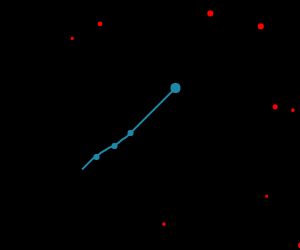# 从零开始开发一款H5小游戏(四) 撞击吧粒子，炫酷技能的实现

@fwon 2016-07-20 02:02:03发表于 fwon/blog

### 碰撞检测

```//index.js
function collision(enemy, player) {
const disX = player.x - enemy.x;
const disY = player.y - enemy.y;

}```

### 撞击敌人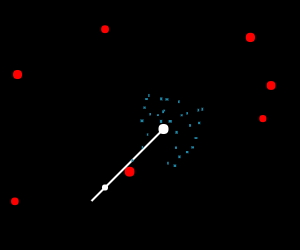Player的闪烁怎么实现呢？如果将这个过程拆解一下，其实闪烁效果就是在一段时间内，Player的颜色不断随机地做蓝白变化。这里只要控制两个变量，闪烁时间和闪烁颜色。

`collision`检测到碰撞的时候，会调用一个flash方法。这个方法有两个作用，一是控制闪烁的时间，通过flashing, 判断是否渲染闪烁效果。二是当时间结束后，我们需要重置Player的颜色为默认的蓝色。

```//Player.js
flash() {
let self = this;

self.flashing = true;
let timeout = setTimeout(function() {
self.flashing = false;
self.color = BODYCOLOR;
clearTimeout(timeout);
}, 500);
}```

```render() {
//闪烁效果
if (this.flashing) {
this.color = ["#fff", BODYCOLOR][Math.round(Math.random())];
}
}```

```//Particle.js
/**
* 爆炸粒子
*/

import map from './Map';

const rand = Math.random;

export default class Particle {

constructor(options) {
this.x = options.x;
this.y = options.y;
this.vx = -2 + 4 * rand();   //速度随机
this.vy = -2 + 4 * rand();   //速度随机
this.destroy = false;
this.speed = 0.04;           //粒子消失的速度
this.size = options.size || 2;
this.color = options.color || "rgb(30,136,168)";
this.width = this.size + rand() * 2; //粒子大小
this.height = this.size + rand() * 2; //粒子大小
}

update() {
//向x轴和y轴的运动
this.x += this.vx;
this.y += this.vy;

//粒子不断变小
this.width -= this.speed;
this.height -= this.speed;

//粒子消失时，将状态至为destroy,不再渲染
if (this.width < 0) {
this.destroy = true;
}
}

render() {
map.ctx.fillStyle = this.color;
map.ctx.fillRect(this.x, this.y, this.width, this.height);
}
}```

```//Player.js
boom(x, y, color, size) {
let self = this;
let eachPartical = [];
for (let i = 0; i < self.particleCount; i++) {
eachPartical.push(new Particle({x, y, color, size}));
}
self.particles.push(eachPartical);
}```

```//Player.js
renderBoom() {
for (let i = 0; i < this.particles.length; i++) {
let eachPartical = this.particles[i];
for (let j = 0; j < eachPartical.length; j++) {
//爆炸粒子消失时，从数组中排除
if (eachPartical[j].destroy) {
eachPartical.splice(j, 1);
} else {
eachPartical[j].render();
eachPartical[j].update();
}
}
}
}

render() {
//爆炸
if (self.particles.length) self.renderBoom();
}```

### 护盾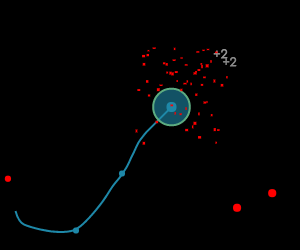```function collision(enemy, player) {
const disX = player.x - enemy.x;
const disY = player.y - enemy.y;
if (player.hasShield) {
}
}```

### 重力场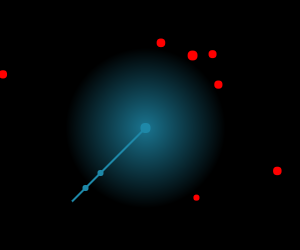```renderGravity() {
map.ctx.beginPath();
map.ctx.globalCompositeOperation="source-over";

map.ctx.arc(this.x, this.y, this.gravityRadius, 0, Math.PI*2, false);
map.ctx.fill();
}```

index.js中，发动机animate方法通过一个循环来渲染Enemy粒子。

```//index.js
function animate() {
for (let i = 0; i < enemys.length; i++) {
enemys[i].render();
enemys[i].update();
if (!player.dead && collision(enemys[i], player)) {
if (player.hasGravity) {
enemys[i].escape(player);
}
}
}
}```

```//Enemy.js
escape(player) {
let ratio = 1/30;
let angle = Math.atan2(this.y - player.y, this.x - player.x);
let ax = Math.abs(player.gravityRadius * Math.cos(angle));
ax = this.x > player.x ? ax : -ax;

let ay = Math.abs(player.gravityRadius * Math.sin(angle));
ay = this.y > player.y ? ay : -ay;

this.vx += ax * ratio;
this.vy += ay * ratio;
this.x += this.vx * ratio;
this.y += this.vy * ratio;
}```

```escape(player) {
let angle = Math.atan(Math.abs(player.y - this.y) / Math.abs(player.x - this.x));

if (this.x > player.x && this.x < player.x + addX) {
this.x += this.speed * 2;
} else if (this.x < player.x && this.x > player.x - addX) {
this.x -= this.speed * 2;
}

if (this.y > player.y && this.y < player.y + addY) {
this.y += this.speed;
} else if (this.y < player.y && this.y > player.y - addY) {
this.y -= this.speed;
}
}```

### 粒子变小&时间变慢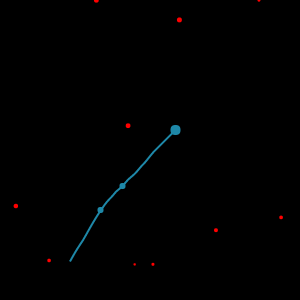### 增加生命